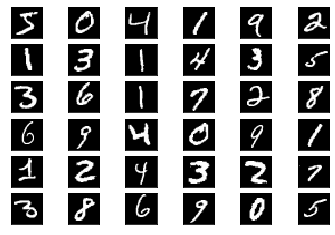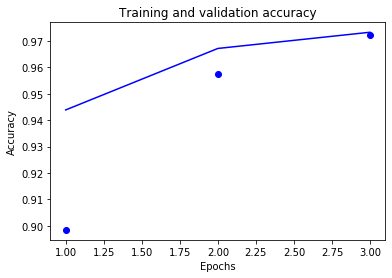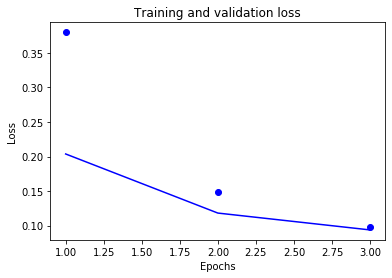## Handwritten Digit Recognition

Objective: Classify handwritten digits from the MNIST dataset by training a convolutional neural network (CNN) using the Keras deep learning library.

In :
``x = 1``

Let's tyr something

In :
``````import os

os.listdir() + [x]
``````
Out:
``['keras-mnist-jovian.ipynb', '.ipynb_checkpoints', 1]``

### Data Preparation

We begin by downloading the data and creating training & validation sets. Keras has inbuilt helper functions to do this.

In :
``````from keras.datasets import mnist
(train_images, train_labels), (test_images, test_labels) = mnist.load_data()
``````
```Using TensorFlow backend. ```

Each sample is a 28px x 28 px image, flattened out a vector of length 784 i.e. 28x28.

In :
``train_images.shape, train_labels``
Out:
``((28, 28), 5)``

Let's take a look at some sample images from the training set, by plotting them in a grid.

In :
``````%matplotlib inline
import matplotlib.pyplot as plt

grid_size = 6
f, axarr = plt.subplots(grid_size, grid_size)
for i in range(grid_size):
for j in range(grid_size):
ax = axarr[i, j]
ax.get_xaxis().set_visible(False)
ax.get_yaxis().set_visible(False)
ax.imshow(train_images[i * grid_size + j], cmap='gray')``````We're going to apply the following preprocessing steps:

1. Reshape the image vectors into 28x28 matrices
2. Separate 20% of the training set to create a vaidation set
3. Conver the numeric labels into one-hot vectors
In :
``````train_images = train_images.reshape((60000, 28, 28, 1))
train_images = train_images.astype('float32') / 255
test_images = test_images.reshape((10000, 28, 28, 1))
test_images = test_images.astype('float32') / 255

from keras.utils import to_categorical

partial_train_images = train_images[:45000]
partial_train_labels = train_labels[:45000]

validation_images = train_images[45000:]
validation_labels = train_labels[45000:]

partial_train_labels = to_categorical(partial_train_labels)
validation_labels = to_categorical(validation_labels)
test_labels = to_categorical(test_labels)
``````

### Model & Training

Now we're ready to define a simple CNN model.

In :
``````input_shape = (28,28,1)
num_classes = 10``````
In :
``````from keras.models import Sequential
from keras.layers import Conv2D, MaxPooling2D, Flatten, Dense

model = Sequential()
model.add(Conv2D(32, kernel_size=(3, 3), activation='relu', input_shape=(28,28,1)))
model.add(MaxPooling2D(pool_size=(2, 2)))
model.add(Flatten())
model.add(Dense(num_classes, activation='softmax'))

model.summary()
``````
```WARNING:tensorflow:From /usr/local/anaconda3/envs/keras-mnist-jovian/lib/python3.7/site-packages/tensorflow/python/framework/op_def_library.py:263: colocate_with (from tensorflow.python.framework.ops) is deprecated and will be removed in a future version. Instructions for updating: Colocations handled automatically by placer. _________________________________________________________________ Layer (type) Output Shape Param # ================================================================= conv2d_1 (Conv2D) (None, 26, 26, 32) 320 _________________________________________________________________ max_pooling2d_1 (MaxPooling2 (None, 13, 13, 32) 0 _________________________________________________________________ flatten_1 (Flatten) (None, 5408) 0 _________________________________________________________________ dense_1 (Dense) (None, 10) 54090 ================================================================= Total params: 54,410 Trainable params: 54,410 Non-trainable params: 0 _________________________________________________________________ ```
In :
``model.compile(optimizer='rmsprop', loss='categorical_crossentropy', metrics=['accuracy'])``
In :
``````import jovian

jovian.log_hyperparams({
'arch': 'CNN(32)+Dense',
'epochs': 3,
'batch_size': 128,
'opt': 'rmsprop'
})
``````
```[jovian] Hypermaters logged. ```
In :
``````history = model.fit(
partial_train_images,
partial_train_labels,
epochs=3,
batch_size=128,
validation_data=(validation_images, validation_labels))
``````
```WARNING:tensorflow:From /usr/local/anaconda3/envs/keras-mnist-jovian/lib/python3.7/site-packages/tensorflow/python/ops/math_ops.py:3066: to_int32 (from tensorflow.python.ops.math_ops) is deprecated and will be removed in a future version. Instructions for updating: Use tf.cast instead. Train on 45000 samples, validate on 15000 samples Epoch 1/3 45000/45000 [==============================] - 14s 314us/step - loss: 0.3799 - acc: 0.8985 - val_loss: 0.2037 - val_acc: 0.9439 Epoch 2/3 45000/45000 [==============================] - 14s 319us/step - loss: 0.1489 - acc: 0.9576 - val_loss: 0.1182 - val_acc: 0.9671 Epoch 3/3 45000/45000 [==============================] - 14s 302us/step - loss: 0.0987 - acc: 0.9724 - val_loss: 0.0938 - val_acc: 0.9733 ```
In :
``````jovian.log_metrics({
'loss': 0.0987,
'acc': 0.9724,
'val_loss': 0.0938,
'val_acc': 0.9733
})
``````
```[jovian] Metrics logged. ```
In :
``````from utils import plot_history

plot_history(history)
``````### Model Evaluation

In :
``test_loss, test_acc = model.evaluate(test_images, test_labels)``
```10000/10000 [==============================] - 1s 116us/step ```
In :
``````print('Test loss:', test_loss)
print('Test acc:', test_acc)``````
```Test loss: 0.07981337974630297 Test acc: 0.976 ```
In :
``````jovian.log_metrics({
'test_loss': 0.07981,
'test_acc': 0.976
})
``````
```[jovian] Metrics logged. ```

We can also save the trained model's weights to disk, so we won't need to train it again.

In :
``model.save('mnist-cnn.h5')``

### Save & Commit

In :
``!pip install jovian --upgrade``
```Collecting jovian Downloading https://files.pythonhosted.org/packages/df/6c/a77a74acf3090f6210ef65799d1cee33fcd3dfb72aa5e52b9ae04c11f71f/jovian-0.1.61-py3-none-any.whl Requirement already satisfied, skipping upgrade: requests in /usr/local/anaconda3/envs/keras-mnist-jovian/lib/python3.7/site-packages (from jovian) (2.22.0) Requirement already satisfied, skipping upgrade: uuid in /usr/local/anaconda3/envs/keras-mnist-jovian/lib/python3.7/site-packages (from jovian) (1.30) Requirement already satisfied, skipping upgrade: certifi>=2017.4.17 in /usr/local/anaconda3/envs/keras-mnist-jovian/lib/python3.7/site-packages (from requests->jovian) (2019.3.9) Requirement already satisfied, skipping upgrade: idna<2.9,>=2.5 in /usr/local/anaconda3/envs/keras-mnist-jovian/lib/python3.7/site-packages (from requests->jovian) (2.8) Requirement already satisfied, skipping upgrade: urllib3!=1.25.0,!=1.25.1,<1.26,>=1.21.1 in /usr/local/anaconda3/envs/keras-mnist-jovian/lib/python3.7/site-packages (from requests->jovian) (1.25.3) Requirement already satisfied, skipping upgrade: chardet<3.1.0,>=3.0.2 in /usr/local/anaconda3/envs/keras-mnist-jovian/lib/python3.7/site-packages (from requests->jovian) (3.0.4) Installing collected packages: jovian Found existing installation: jovian 0.1.60 Uninstalling jovian-0.1.60: Successfully uninstalled jovian-0.1.60 Successfully installed jovian-0.1.61 ```
In :
``import jovian``
In [ ]:
``jovian.commit(files=['utils.py'], artifacts=['mnist-cnn.h5'])``
```[jovian] Saving notebook.. ```
In [ ]:
`` ``